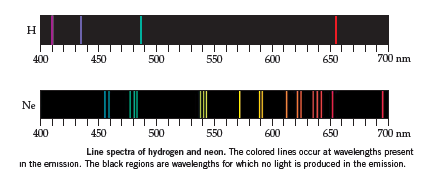# Problem: In the top part of image below, the four lines in the H atom spectrum are due to transitions from a level for which ni &gt; 2 to the nf = 2 level. What is the value of ni for the red line in the spectrum?

###### FREE Expert Solution

We’re being asked to determine the value of nI

We’re going to use the Balmer Equation which relates wavelengths to a photon’s electronic transitions.

$\overline{)\frac{\mathbf{1}}{\mathbf{\lambda }}{\mathbf{=}}{{\mathbf{RZ}}}^{{\mathbf{2}}}\left(\frac{\mathbf{1}}{{{\mathbf{n}}^{\mathbf{2}}}_{\mathbf{final}}}\mathbf{-}\frac{\mathbf{1}}{{{\mathbf{n}}^{\mathbf{2}}}_{\mathbf{initial}}}\right)}$

λ = wavelength, m
R = Rydberg constant = 1.097x107 m-1
Z = atomic number of the element
ninitial = initial energy level
nfinal = final energy level

Calculate the initial energy level (ninitial):

96% (291 ratings)###### Problem Details

In the top part of image below, the four lines in the H atom spectrum are due to transitions from a level for which ni > 2 to the nf = 2 level. What is the value of ni for the red line in the spectrum?Frequently Asked Questions

What scientific concept do you need to know in order to solve this problem?

Our tutors have indicated that to solve this problem you will need to apply the Bohr Equation concept. You can view video lessons to learn Bohr Equation. Or if you need more Bohr Equation practice, you can also practice Bohr Equation practice problems.

What professor is this problem relevant for?

Based on our data, we think this problem is relevant for Professor Carra's class at HCC.